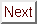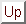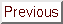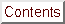Next: Dimensional Crossover Models Up: Upper Critical Field Previous: Experimental Results: Temperature

# Possible Interpretations of Enhanced Linearity and Positive Curvature in GIC's

This section is not intended to be a complete review of Hc2(T) theories. Instead, the following is a discussion of a few specific models which predict enhanced critical fields and the prospects for the application of these models to GIC's. A recent complete overview of Hc2(T) theories has been written by Decroux and Fischer.

Before reviewing the models which help to explain the deviations from the anisotropic Ginzburg-Landau theory, it makes sense to review the basic AGL model itself. The anisotropic Ginzburg-Landau model, like the Ginzburg-Landau theory from which it is descended, is based on an expansion of the free-energy difference between the superconducting and normal phases into a series of powers of the order parameter. For superconductivity, the order parameter is variously taken as the energy gap (in theories based on the Gor'kov equations[94,93]), the density of superfluid particles (in the spirit of the two-fluid model), or the Ginzburg-Landau wave function psi. In the simplest case of the isotropic GL theory, the free-energy difference of the superconducting and normal states is written:Here alpha is the kinetic energy of the Cooper pair, and vecA is the vector potential. The GL differential equations are derived from the free-energy variationally. Their solution for the zero-field case shows that | alpha | = hbar2 / 2m* xi2, where xi is the superconducting coherence length discussed in Section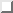. Using the relation Hc2 = ø0 / 2pi xi 2 and rearranging factors shows that alpha is equal to the cyclotron energy of an electron at H = Hc2. This equality points to the connection between vortices in type II superconductors and the Landau-level problem which is mentioned in Tinkham's book.[252, Section 4-8,]

The only elaboration necessary to go from the isotropic to the anisotropic case is to let the mass m* become a tensor rather than a scalar.[244,127] All the rest of the results of the AGL model follow directly from this replacement. The spirit of the AGL model is to let all the orientation dependence be lumped into the masses, and to ignore any variation with angle of other microscopic parameters (e.g., mean free path and phonon dispersion). Therefore, all anisotropy effects are described in this model by one temperature-independent parameter, epsilon. This parameter was introduced by Morris, Coleman, and Bhandari who defined epsilon == sqrtm/M, where m is the in-plane mass and M is the c-axis mass. With the assumption of in-plane isotropy, further calculation shows that Hc2, || ^c/Hc2, _|_ ^c = xi_|_ ^c/xi|| ^c. Because epsilon is the sum of all the contributions to the anisotropy of a layered superconductor, it should not necessarily be thought of as the ratio of band masses. Similarly, the fact that the flux quantum in the AGL model is ellipsoidal does not imply the presence of an ellipsoidal Fermi surface.

The simplicity of the AGL model is both its greatest virtue and its greatest weakness. On one hand, the small number of parameters in the model allow calculation of quite complex quantities, such as the angular dependence of the lower critical field, Hc1. No sane person would seriously contemplate the calculation of Hc1(theta) in the context of a first-principles model of anisotropic superconductivity, where closed-form final expressions are rare. On the other hand, the AGL model is so simple that it is inadequate to describe all the details of superconductivity in GIC's, despite the fact that it gives a satisfactory picture of the main features. Both the poor fits to Hc2(theta) (see Section) and the temperature dependence of epsilon (see Section) cannot be explained in the context of the AGL model. Furthermore, the enhanced linearity of Hc2(T) cannot be explained within the WHH theory, which reduces to the AGL model for T Tc.[94,84] Considering the many aspects of anisotropy in GIC's, it is not surprising that the one parameter epsilon is insufficient to summarize all their manifestations. All in all, the anisotropic Ginzburg-Landau model is successful within the confines of its applicability, and useful as far as the physical insight it provides, but it is not the last word on the subject by any means.

Before going into which models are the most appropriate for a detailed application to C4KHg, it is useful to quickly discuss those possibilities which produce similar effects in the data, but are not applicable here. Most of these models are extensions of the WHH theory, but one, the Klemm-Luther-Beasley (KLB) dimensionality-crossover model, is based more directly on the AGL model.Next: Dimensional Crossover Models Up: Upper Critical Field Previous: Experimental Results: Temperature

alchaiken@gmail.com (Alison Chaiken)
Wed Oct 11 22:59:57 PDT 1995